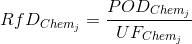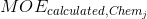# Cumulative Risk Characterization

Cumulative exposure is defined as the exposure to multiple chemicals from multiple pathways acting through a common mechanism of toxicity.
The first step in performing a cumulative risk assessment is to identify a common mechanism group. This involves identifying pesticides that share a common mode of action.
Once a group of pesticides with a common mode of action is selected, the toxic potencies for the common effect are estimated. The user will be required to input a set of Reference Doses (RfDs) for his/her set of pesticides and to identify an Index Chemical from the set. The purpose of the Index Chemical is to provide a point of reference from which the toxic potencies for all chemicals within the group can be standardized. The software will then calculate a set of Relative Potency Factors (RPFj) across the selected pesticides j=1,…,M as per the following formula.Whereis the Reference Dose,the Point of Departure andthe Uncertainty Factor of the j-th pesticide.
The RPFj is multiplied by the exposure to chemical j,, to determine the Exposure Equivalents in terms of the Index Chemical. The Cumulative exposure is the sum of all the Exposure Equivalents for each chemical in the common mechanism group. RPFj will be the basis of cumulative exposure assessments and the cumulative exposure will be calculated as per the following formula:where M is the number of pesticides under analysis.

Analogously with aggregate exposure, calculations on the Cumulative Margin of Exposure, Cumulative Risk Index, and Cumulative Hazard Index are required; the Cumulative MOE will be the default calculation.
The co-exposure information for each sample within the monitoring data, in particular the data obtained from the PDP database, are preserved and used in the cumulative assessment for multiple chemicals.

# Cumulative margin of exposurewhereand Chemj represents j-th chemical of the M analysed ones. The Point of Departure (mg/kg/day) may be the NOAEL, ED10 or other relevant measure of the toxicological endpoint describing the common mechanism of effect, and the Dose is the estimated dose (mg/kg/day) from the model for the specified chemical.
In the assessments where only a single chemical is analysed, the CARES NG software will calculate the MOE of that chemical and the MOECumulative will be set equal to the MOE of the analysed chemical.

# Cumulative risk index

The Cumulative Risk Index (CRI) formula is based on the Aggregate Risk Index (ARI) and is used when multiple MOEs must be cumulated, but different uncertainty factors have been applied to each individual MOE.whereTheis the Margin of Exposure incorporating the uncertainty factor(s) specified by the user and/or regulatory agency and thecomes from equation (1) for the specified chemical. The ARI was designed to incorporate into a single index each route’s potency when route-specific Points of Departure are used.